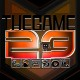Enter the Infinite Rabbit HoleC o l l a g e c r e a t e d b y m y s e l f a n d w h i c h I t i t l e d … “ 𝑫𝒂𝒅 𝒘𝒊𝒕𝒉 𝒉𝒊𝒔 𝒄𝒉𝒊𝒍𝒅𝒓𝒆𝒏 ”

C o l l a g e c r e a t o d a l s o t t o s c r i t t o e c h e h o i n t i t o l a t o … “ 𝑫𝒂𝒅 𝒘𝒊𝒕𝒉 𝒉𝒊𝒔 𝒄𝒉𝒊𝒍𝒅𝒓𝒆𝒏 ”